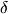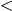## Cohen, Haeupler, and Schulman: Explicit Binary Tree-Codes & Cancellations

The high-dimensional conference in Jerusalem is running with many exciting talks (and they are videotaped), and today in Tel Aviv there is a conference on Optimization and Discrete Geometry : Theory and Practice.

Today in Jerusalem, Leonard Schulman talked (video available!) about a  recent breakthrough by  Gil Cohen, Bernhard Haeupler, and Leonard Schulman in the recent paper Explicit Binary Tree Codes with Polylogarithmic Size Alphabet.

## Tree codes

Tree codes are extremely important objects invented by Leonard Schulman in 1992-1996. You can read about it in Wigderson’s book  Mathematics and Computation  (see also this post) in a whole section about  Error-correction of interactive communication, a theory pioneered by Leonard. Finding explicit good (linear distance, finite alphabet) tree codes is a very important problem.

(From the introduction of the new paper) “A tree code consists of a complete rooted binary tree (either infinite or of finite depth$n$) in which each edge is labeled by a symbol from an alphabet$\Sigma$ . There is a natural one-to-one mapping assigning each binary string$s$ to a path starting at the root, where$s$ simply indicates which child is taken in each of the steps. For a tree code, such a path naturally maps to a string over the alphabet$\Sigma$, which is formed by concatenating the symbols along the path. This way a tree code$T$ encodes any binary string$s$ into an equally long string$T(s)$ over$\Sigma$. This encoding has an online characteristic because the encoding of any prefix does not depend on later symbols. In particular, any two distinct strings that agree in their first$k$ symbols also have encodings that agree in their first$k$ symbols. A tree code is said to achieve distance$\delta \ge 0$ if the encodings of any two strings differ in at least a$\delta$-fraction of the positions after their first disagreement. The rate of a tree code is$1/\log _2 (\Sigma )$. A tree code is said to be asymptotically good if it achieves both constant distance$\delta >0$  and a constant rate, namely, the alphabet size is$O(1)$.”

### The abstract:

“This paper makes progress on the problem of explicitly constructing a binary tree code with constant distance and constant alphabet size.

For every constant1 we give an explicit binary tree code with distanceand alphabet size$(\log n)^{O(1)}$, where n is the depth of the tree. This is the first improvement over a two-decade-old construction that has an exponentially larger alphabet of size$n^{O(1)}$.

As part of the analysis, we prove a bound on the number of positive integer roots a real polynomial can have in terms of its sparsity with respect to the Newton basis – a result of independent interest.”

### Problems on Exponential sums

A few years ago Cris Moore and Leonard Schulman proposed an explicit constructions for good tree codes in the paper Tree codes and a conjecture on exponential sums. The construction depends on still open conjectures on new types of exponential sums.

## A paper by Leonard and me on polynomials with massive cancellations.

So let me use this opportunity to advertize a paper by Leonard and me Quasi-random multilinear polynomials which was just accepted for publication in the Israel Journal of Mathematics. Unexpected cancellation is always a very interesting phenomenon that we cherish and wish to understand. For example, we expect that the sum of the Mobius function for the first n integers cancels almost like that of a random walk. Depending on the meaning of “almost” this is the (known) prime number theorem, the (conjectured) Riemann hypothesis, or simply false, respectively.

A little less famous example  (which I personally like) is the paper by Nikola Djokic  an upper bound on the sum of signs of permutations with a condition on their prefix sets solving a problem by J. Feldman, H. Knorrer, and E. Trubowitz.

Our starting point is the determinant: It is a polynomial of degree$n$ of$n^2$ variables and no matter what$\pm 1$ values you assign to the variables you have a massive cancellation of almost a random-walk type. There are$n!$ terms and for any assignment the value is smaller than$n^{n/2}$ which is$(n!)^{1/2+o(1)}$.  We were interested in the question of finding other such polynomials.

This entry was posted in Combinatorics, Computer Science and Optimization, Open problems and tagged , , , . Bookmark the permalink.

### 2 Responses to Cohen, Haeupler, and Schulman: Explicit Binary Tree-Codes & Cancellations

1.arielgabizon says:

Loved the result on tree codes. Typo ‘delta’ instead of symbol delta.

GK: many thanks Ariel